Latest SSC jobs   »   Quantitative Aptitude Quiz SSC CHSL 4th...

# Quantitative Aptitude Quiz SSC CHSL 4th February 2020 For Average ,Percentage

Q1. A can do 75% of a job in 18 days and B can do 25% of the job in 12 days. If they work on it together, in how many days can they do 75% of the job?
(a) 16
(b) 8
(c) 20
(d) 12

Q2. Area of 4 walls of a cuboid is 57 sq m, if its length is 5.5 m and height is 3 m. What is its breadth (in m)?
(a) 4.5
(b) 4
(c) 3
(d) 3 .5

Q3. At 30% discount the selling price of an article is Rs 1050. What is the selling price (in Rs) if the discount is 15%?
(a) 1200
(b) 1175
(c) 1100
(d) 1275

Q4. What is the fourth proportional to 336, 288 and 161?
(a) 184
(b) 115
(c) 138
(d) 134

Q5. What is the average of all numbers between 9 and 90 which are divisible by 8?
(a) 53
(b) 52
(c) 51
(d) 50

Q6. A trader had 630 kgs of rice. He sold a part of it at 15% profit and the rest at 8% profit, so that he made a total profit of 12%. How much rice (in kgs)
did he sell at 8% profit?
(a) 270
(b) 300
(c) 280
(d) 290

Q7.50% of a = b, then b% of 40 is the same as _________ of a.
(a) 0.25
(b) 0.16
(c) 2
(d) 0 .2

Q8. Excluding stoppages, the speed of a train is 120 kmph and including stoppages, it is 50 kmph. For how many minutes does the train stop per hour?
(a) 25
(b) 40
(c) 35
(d) 20

Q9. The simple and compound interest that can be earned in two years at the same rate is Rs 4000 and Rs 4180 respectively. What is the rate (percent per annum) of interest?
(a) 18
(b) 4.5
(c) 9
(d) 1 2

Q10. If 2x/3 – [5(4x/5 – 4/3)]/2 = 1/3, then what is the value of x?
(a) 9/4
(b) 4/9
(c) -9/4
(d) -4/9

#### Solutions: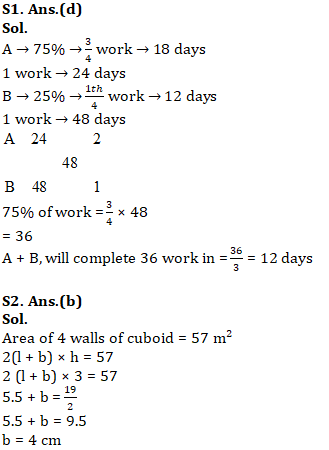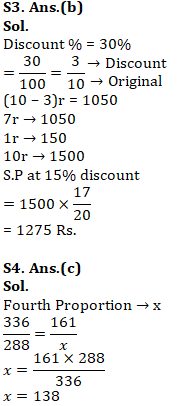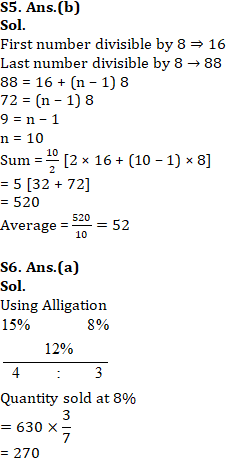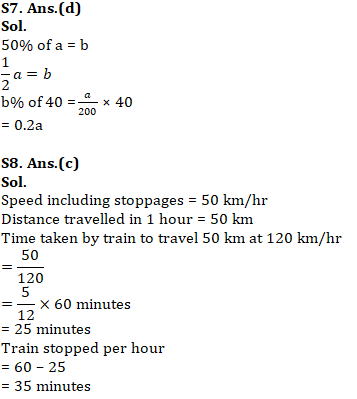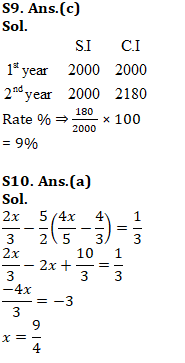You may also like to read:

#### Congratulations!General Awareness & Science Capsule PDF